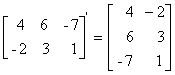Left Border Content - RF CafeAbout RF CafeCopyright: 1996 - 2024Webmaster:    Kirt Blattenberger,     BSEE - KB3UON RF Cafe began life in 1996 as "RF Tools" in an AOL screen name web space totaling 2 MB. Its primary purpose was to provide me with ready access to commonly needed formulas and reference material while performing my work as an RF system and circuit design engineer. The World Wide Web (Internet) was largely an unknown entity at the time and bandwidth was a scarce commodity. Dial-up modems blazed along at 14.4 kbps while typing up your telephone line, and a nice lady's voice announced "You've Got Mail" when a new message arrived... All trademarks, copyrights, patents, and other rights of ownership to images and text used on the RF Cafe website are hereby acknowledged. My Hobby Website: AirplanesAndRockets.comSub-Header - RF Cafe# Matrix Definitions

In mathematics, a matrix (plural matrices, or less commonly matrixes) is a rectangular array of numbers, as shown at the right. One use of matrices is to keep track of the coefficients in a system of linear equations. Matrices can also represent linear transformations, which are higher-dimensional analogs of linear functions of the form f(x) = cx, where c is a constant. They can be added and subtracted entrywise, and multiplied according to a rule corresponding to composition of linear transformations. These operations satisfy the usual identities, except that matrix multiplication is not commutative: the identity AB=BA can fail. For a square matrix, the determinant and inverse matrix (when it exists) govern the behavior of solutions to the corresponding system of linear equations, and eigenvalues and eigenvectors provide insight into the geometry of the associated linear transformation.

Matrices find many applications. Physics makes use of them in various domains, for example in geometrical optics and matrix mechanics. The latter also led to studying in more detail matrices with an infinite number of rows and columns. Matrices encoding distances of knot points in a graph, such as cities connected by roads, are used in graph theory, and computer graphics use matrices to encode projections of three-dimensional space onto a two-dimensional screen. Matrix calculus generalizes classical analytical notions such as derivatives of functions or exponentials to matrices. The latter is a recurring need in solving ordinary differential equations. Serialism and dodecaphonism are musical mouvements of the 20th century that utilize a square mathematical matrix (music) to determine the pattern of music intervals. - Wikipedia

 Unity Elementary SymmetricDiagonal Matrix with maindiagonal elements = 1All elements = 0 exceptfor  a single 1Square Matrix withaij = aji Square Diagonal Null Same number of rows and columnsSquare Matrix withaij = 0 for all i¹ jAll elements = 0 Singular Value of determinant = 0

 DeterminantAdjointSquare Matrix where aij is replaced with I, j cofactor multiplied by (-1)i·j. Adjoint A = adj A Rank Maximum number of linearly independent columns of the matrix A. Order of the longest nonsingular matrix contained in matrix A. Rank of A = Rank of A'     Rank of A = Rank of A'A   Rank of A = Rank of A A' TransposeMatrix where rows and columns are interchanged.Transpose A = A(A')' = A(kA)' = kA' (A+B)' = A'+B'(AB)' = B'A'
Footer - RF CafeRight Border Content - RF CafePlease Support RF Cafe by purchasing my  ridiculously low−priced products, all of which I created. These Are Available for Free